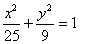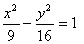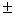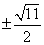Monday 08th August 2022CBSE Guess > Papers > Important Questions > Class XI > 2010 > Maths > Maths By Mr. Anil Kumar Tondak CBSE CLASS XI
Conic Sections Q.1. Find the centre and radius of the circle 2x2 + 2y2 –x = 0. Q.2. Find the equation of the circle with radius 5 whose centre lies on x-axis and passes through the point (2,3). Q.3. Find the equation of the circle passing through the points (2,3) and (–1,1) and whose centre is on the line x – 3y – 11 = 0. Q.4. Find the equation of the circle passing through (0,0) and making intercepts a and b on the coordinate axes. Q.5. Find the equation of the parabola with vertex at (0, 0) and focus at (0, 2). Q.6 . Find the equation of the parabola with focus (2,0) and directrix x = – 2. Q.7. Find the equation of the parabola which is symmetric about y-axis and passes through the point (2, -3). Q.8. Find the coordinates of the foci, the vertices, the length of major axis, the minor axis, the eccentricity and the latus rectum of the ellipse :Q.9. Find the co-ordinates of the foci, the vertices, the lengths of major and minor axes and the eccentricity of the ellipse 9x2 + 4y2 = 36. Q.10. Find the equation of the ellipse with major axis along the x-axis and passing through the points (4, 3) and (-1, 4). Q.11. Find the equation of the ellipse, whose length of the major axis is 20 and foci are (0, ± 5). Q12. Find the coordinates of the foci and the vertices, the eccentricity,the length of the latus rectum of the hyperbolas: (i)(ii) y2 – 16x2 = 1 Q.13. Find the equation of the hyperbola with foci (0,3) and vertices (0,). Q.14. Find the equation of the hyperbola with vertices (7,0) and e = 4/3. Q.15. Find the equation of the hyperbola where foci are (0, ±12) and the length of the latus rectum is 36. Q.16. A beam is supported at its ends by supports which are 12 metres apart. Since the load is concentrated at its centre, there is a deflection of 3 cm at the centre and the deflected is in the shape of a parabola. How far from the centre is the deflection 1 cm. Paper By Mr. Anil Kumar Tondak Email Id : [email protected] Ph No.: 9811363962